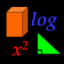iMathSchool - Slunečnice.cz Hlavní navigace

#iMathSchool 2.3

3

Pro hodnocení programu se prosím nejprve

Staženo 0 ×
Cena: 0,99 €

## Sdílet

This application is a simple intuitive calculator that has almost all the functions of a scientific calculator but with an attractive and didactic interface. With it you can compute all trigonometric functions and their inverses. Calculate the perimeter, area and volume of common geometric figures. Get the inverse and the determinant of 2x2 and 3x3 matrices. Convert units of temperature, weight, length, area and volume of various metric systems. Calculate natural logarithms and anti-logarithms of different bases. Calculate roots and powers. Solve quadratic equations and systems of linear equations 2x2 and 3x3. And make arithmetic operations with fractions. It will be very useful for high school students.

2.3

• #### Poslední aktualizace od vývojáře

7. 3. 2017 2017-03-07

0 ×

• #### Velikost

3,31 MB 3.31 MB

• Vzdělávání